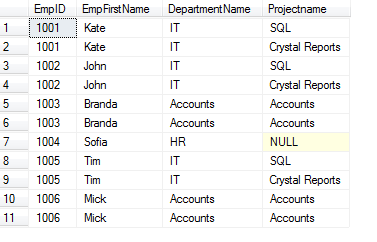# Cross Apply And Outer Apply With Examples

This article describes Cross Apply and Outer Apply and gives some examples with functions.

The Apply operator joins two table valued expressions, the table on right is evaluated every time for each row of the table on the left which is actually a table-valued function. The final outcome contains all the selected columns from the left side table and then from the right side table.

1. Cross Apply
2. Outer Apply

1. ## CROSS APPLY

The Cross Apply returns rows from the outer table (table on the left of the Apply operator) that produces matching values from the table-valued function (which is on the right side of the operator).

The Cross Apply is equivalent to Inner Join, but it works with a table-valued function.

Example: To view the working of the Cross Apply operator first we shall create two tables namely EmployeeDetails and EmpSalary.

Below is the schema and create a query for EmployeeDetails

```    CREATE TABLE EmployeeDetails (
EmpId int PRIMARY KEY,
EmpFirstName VARCHAR(50),
EmpLastName VARCHAR(50),
Department VARCHAR(50),
DepartID INT
)
```

The data in EmployeeDetails table is provided below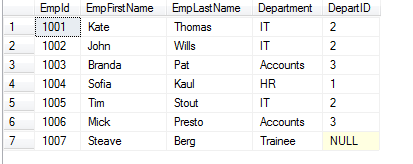Now Create another table EmpSalary

```    CREATE TABLE EmpSalary (
EmpID INT,
EmpFullName VARCHAR(80),
EmpSalary INT,
EmpWorkingYears INT,
DepartID INT
)
```

Here is the data in EmpSalary tableNow we will create a user defined function of SQL server with the name fn_Salaryinc, this function returns output with increased salary by Rs.5000 on the basis of DepartID column.

```    CREATE FUNCTION fn_Salaryinc (@DepartmentID int)
RETURNS TABLE
AS
RETURN
(
SELECT
EmpID, EmpFullName,
EmpSalary+5000 AS Salaryinc
FROM
Empsalary
WHERE
DepartID = @DepartmentID

)
GO

```

Use funtion fn_Salaryinc to get increased salary.

```    SELECT EmpID, Salaryinc FROM fn_Salaryinc(2)
```

After applying the above function the salary got increased by 5000. The below table shows the output for the function fn_SalaryincNow since the function is giving the desired results we will write a Cross apply Query

```    SELECT
e.EmpFirstName,
e.EmpLastName,
f.Salaryinc
FROM
EmployeeDetails AS e
CROSS APPLY
fn_Salaryinc  (e.DepartID) AS f

```

The output of the above query which shows the functioning for Cross Apply operator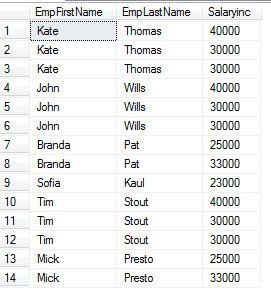We are getting repetitive employees as we are running the function, and it gets data once for each Departid.

2. ## OUTER APPLY

The Outer Apply returns all the rows from the outer table (table on the left of the Apply operator), and rows that do not match the condition from the table-valued function (which is on the right side of the operator), NULL values are displayed.

The Outer Apply is equivalent to Left Outer Join, but it works with a table-valued function.

Example: First create a table EmployeeDetails with the below given query

```
CREATE TABLE EmployeeDetails (
EmpId int PRIMARY KEY,
EmpFirstName VARCHAR(50),
EmpLastName VARCHAR(50),
Department VARCHAR(50),
DepartID INT
)
```

Here is the EmployeeDetails dataCreate another table EmployeeProject

```     CREATE TABLE EmployeeProject (
EmpID INT,
DepartmentName VARCHAR(100),
DepartID INT,
ProjectName VARCHAR(100),
Projectid VARCHAR(50)
)
```

The data for this table is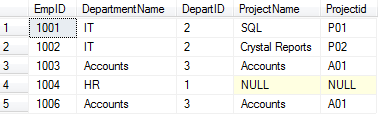Let's create a function named fn_Project, the function returns the column from EmployeeProject table after producing DepartID as a parameter

```    CREATE FUNCTION fn_Project(@DepartID INT)
RETURNS TABLE
AS
RETURN
(
SELECT
EmpID,
DepartmentName,
ProjectName
FROM
EmployeeProject
WHERE
DepartID = @DepartID
)
GO

```

Run the below given query to verify the results

```    SELECT  * FROM fn_Project(2)
```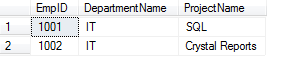Now we will write an Outer Apply Query in order to check the functioning and the results of the operator.

```    SELECT
e.EmpID,
e.EmpFirstName ,
fn.DepartmentName,
fn.Projectname
FROM
Employeedetails e
OUTER APPLY
fn_Project (e.departID) AS fn

```

The Outer Apply query gives the result as shown below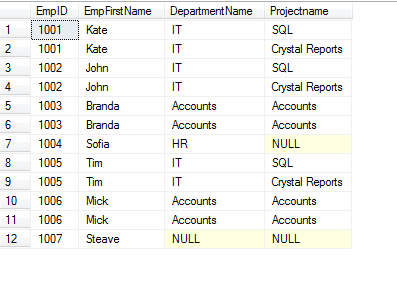Hence by using the Outer Apply operator we are able to return all the rows from the outer table no matter if the function returns any row.

## Difference between Outer Apply and Cross Apply

Let me show you another example using the same above Outer Apply query with Cross Apply, it will clearly show the difference as the number of rows will be lesser as per the join condition.

```    SELECT
e.EmpID,
e.EmpFirstName,
fn.DepartmentName,
fn.Projectname
FROM
Employeedetails e
Cross APPLY
fn_Project (e.departID) AS fn

```